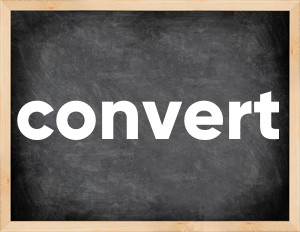# Convert past tenseThe English verb 'convert' is pronounced as [kənˈvɜːrt].
Related to: regular verbs.
3 forms of verb convert: Infinitive (convert), Past Simple - (converted), Past Participle - (converted).

## Here are the past tense forms of the verb convert

👉 Forms of verb convert in future and past simple and past participle.
❓ What is the past tense of convert.

## Convert: Past, Present, and Participle Forms

Base Form Past Simple Past Participle
convert [kənˈvɜːrt]

converted [kənˈvɜːtɪd]

converted [kənˈvɜːtɪd]

## What are the 2nd and 3rd forms of the verb convert?

🎓 What are the past simple, future simple, present perfect, past perfect, and future perfect forms of the base form (infinitive) 'convert'?

### Learn the three forms of the English verb 'convert'

• the first form (V1) is 'convert' used in present simple and future simple tenses.
• the second form (V2) is 'converted' used in past simple tense.
• the third form (V3) is 'converted' used in present perfect and past perfect tenses.

## What are the past tense and past participle of convert?

The past tense and past participle of convert are: convert in past simple is converted, and past participle is converted.

### What is the past tense of convert?

The past tense of the verb "convert" is "converted", and the past participle is "converted".

### Verb Tenses

Past simple — convert in past simple converted (V2).
Future simple — convert in future simple is convert (will + V1).
Present Perfect — convert in present perfect tense is converted (have/has + V3).
Past Perfect — convert in past perfect tense is converted (had + V3).

### convert regular or irregular verb?

👉 Is 'convert' a regular or irregular verb? The verb 'convert' is regular verb.

## Examples of Verb convert in Sentences

•   He can convert many people to Christianity. (Present Simple)
•   We converted 2 flats into an office. (Past Simple)
•   15 servers were converted to virtual machines last week. (Past Simple)
•   How much does it cost today to convert rubles into dollars? (Present Simple)
•   I succeeded in converting my teacher to my point of view. (Past Simple)
•   They converted the spare bedroom into a cinema. (Past Simple)
•   I had a chance to score but I couldn't convert. (Past Simple)
•   He hoped to convert his party members to a belief in communism. (Past Simple)
•   Will it convert the energy of the nuclear reactor into microwaves? (Future Simple)
•   We will convert this old house into a modern one. (Future Simple)

Along with convert, words are popular spoil and let.

Verbs by letter: , , , , , , , , , , , , , , , , , , , , , , , , .